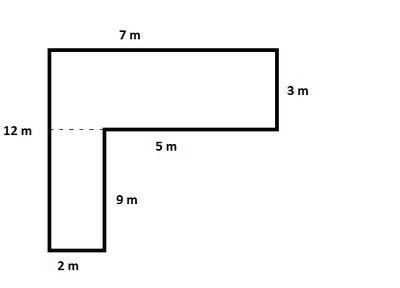## Basic College Mathematics (9th Edition)

$P$ = 38 m $A$ = 39 m$^{2}$
To find the perimeter add all of the length of sides together. $P$ = 7 m + 3 m + 5 m + 9 m + 2 m + 12 m $P$ = 38 m To find the area we can break the shape into two rectangles. The first measuring 7 m x 3 m and the second measuring 9 m x 2 m. Find the area of each and then add the results together to determine the total area. $A_{1}$ = 7 m * 3 m $A_{1}$ = 21 m$^{2}$ $A_{2}$ = 9 m * 2 m $A_{2}$ = 18 m$^{2}$ $A$ = A$_{1}$ + A$_{2}$ $A$ = 21 m$^{2}$ + 18 m$^{2}$ $A$ = 39 m$^{2}$